# Crystal structure  (Page 7/9)

 Page 7 / 9

## Diamond cubic

The diamond cubic structure consists of two interpenetrating face-centered cubic lattices, with one offset 1 / 4 of a cube along the cube diagonal. It may also be described as face centered cubic lattice in which half of the tetrahedral sites are filled while all the octahedral sites remain vacant. The diamond cubic unit cell is shown in [link] . Each of the atoms (e.g., C) is four coordinate, and the shortest interatomic distance (C-C) may be determined from the unit cell parameter ( a ).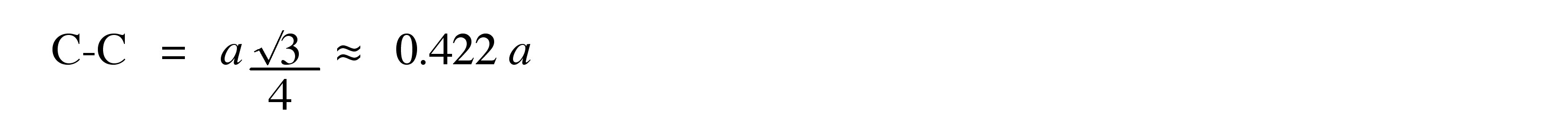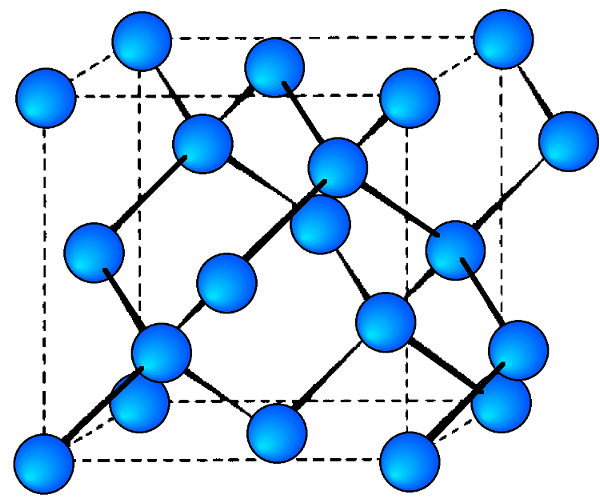Unit cell structure of a diamond cubic lattice showing the two interpenetrating face-centered cubic lattices.

## Zinc blende

This is a binary phase (ME) and is named after its archetype, a common mineral form of zinc sulfide (ZnS). As with the diamond lattice, zinc blende consists of the two interpenetrating fcc lattices. However, in zinc blende one lattice consists of one of the types of atoms (Zn in ZnS), and the other lattice is of the second type of atom (S in ZnS). It may also be described as face centered cubic lattice of S atoms in which half of the tetrahedral sites are filled with Zn atoms. All the atoms in a zinc blende structure are 4-coordinate. The zinc blende unit cell is shown in [link] . A number of inter-atomic distances may be calculated for any material with a zinc blende unit cell using the lattice parameter ( a ).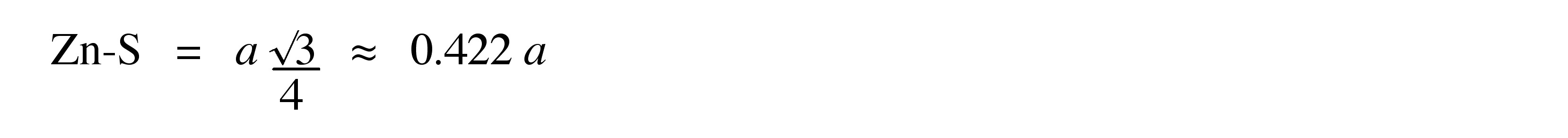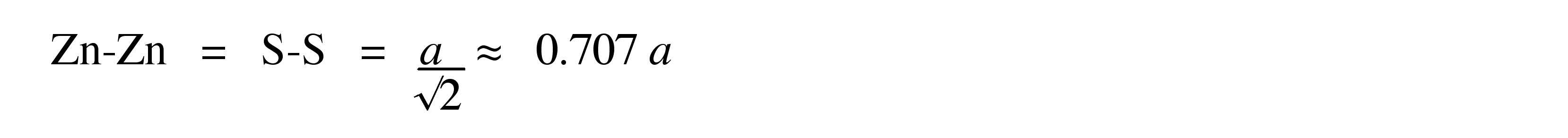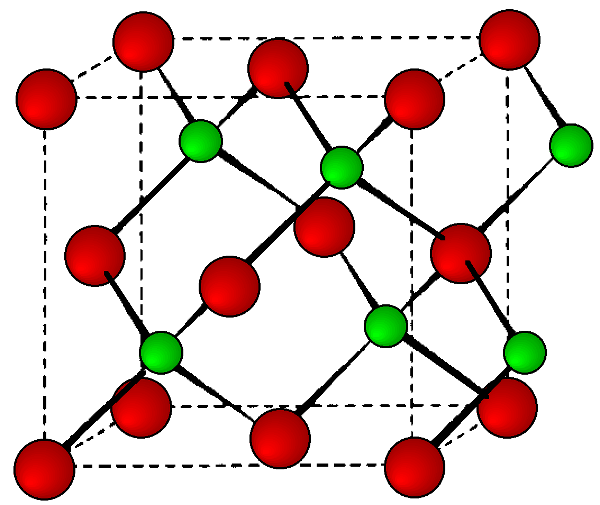Unit cell structure of a zinc blende (ZnS) lattice. Zinc atoms are shown in green (small), sulfur atoms shown in red (large), and the dashed lines show the unit cell.

## Chalcopyrite

The mineral chalcopyrite CuFeS 2 is the archetype of this structure. The structure is tetragonal ( a = b c , α = β = $\gamma$ = 90°, and is essentially a superlattice on that of zinc blende. Thus, is easiest to imagine that the chalcopyrite lattice is made-up of a lattice of sulfur atoms in which the tetrahedral sites are filled in layers, ...FeCuCuFe..., etc. ( [link] ). In such an idealized structure c = 2 a , however, this is not true of all materials with chalcopyrite structures.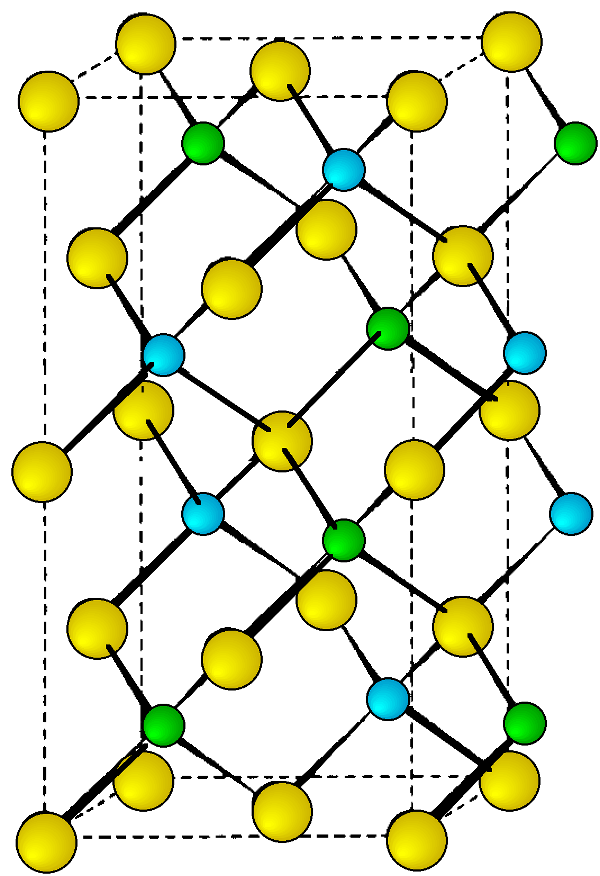Unit cell structure of a chalcopyrite lattice. Copper atoms are shown in blue, iron atoms are shown in green and sulfur atoms are shown in yellow. The dashed lines show the unit cell.

## Rock salt

As its name implies the archetypal rock salt structure is NaCl (table salt). In common with the zinc blende structure, rock salt consists of two interpenetrating face-centered cubic lattices. However, the second lattice is offset 1/2 a along the unit cell axis. It may also be described as face centered cubic lattice in which all of the octahedral sites are filled, while all the tetrahedral sites remain vacant, and thus each of the atoms in the rock salt structure are 6-coordinate. The rock salt unit cell is shown in [link] . A number of inter-atomic distances may be calculated for any material with a rock salt structure using the lattice parameter ( a ).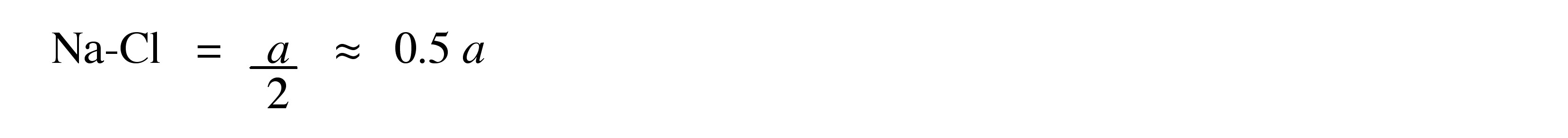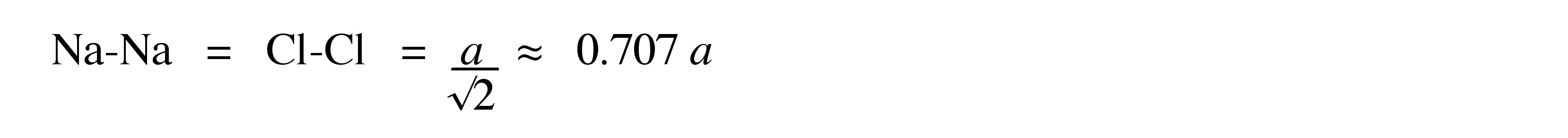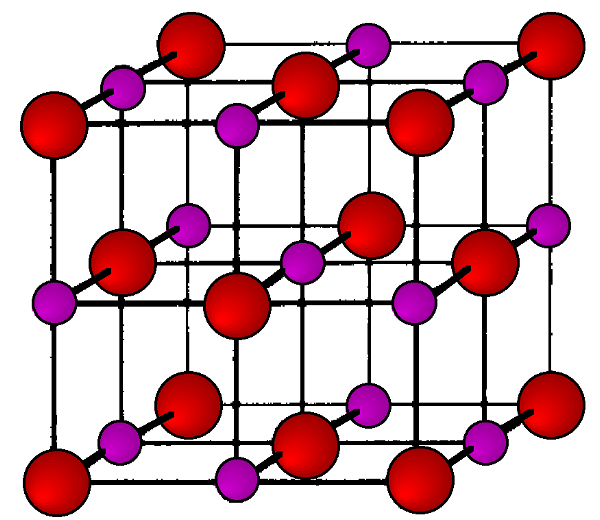Unit cell structure of a rock salt lattice. Sodium ions are shown in purple (small spheres) and chloride ions are shown in red (large spheres).

## Cinnabar

Cinnabar, named after the archetype mercury sulfide, HgS, is a distorted rock salt structure in which the resulting cell is rhombohedral (trigonal) with each atom having a coordination number of six.

find the equation of the line if m=3, and b=-2
graph the following linear equation using intercepts method. 2x+y=4
Ashley
how
Wargod
what?
John
ok, one moment
UriEl
how do I post your graph for you?
UriEl
it won't let me send an image?
UriEl
also for the first one... y=mx+b so.... y=3x-2
UriEl
y=mx+b you were already given the 'm' and 'b'. so.. y=3x-2
Tommy
Please were did you get y=mx+b from
Abena
y=mx+b is the formula of a straight line. where m = the slope & b = where the line crosses the y-axis. In this case, being that the "m" and "b", are given, all you have to do is plug them into the formula to complete the equation.
Tommy
"7"has an open circle and "10"has a filled in circle who can I have a set builder notation
x=-b+_Гb2-(4ac) ______________ 2a
I've run into this: x = r*cos(angle1 + angle2) Which expands to: x = r(cos(angle1)*cos(angle2) - sin(angle1)*sin(angle2)) The r value confuses me here, because distributing it makes: (r*cos(angle2))(cos(angle1) - (r*sin(angle2))(sin(angle1)) How does this make sense? Why does the r distribute once
so good
abdikarin
this is an identity when 2 adding two angles within a cosine. it's called the cosine sum formula. there is also a different formula when cosine has an angle minus another angle it's called the sum and difference formulas and they are under any list of trig identities
strategies to form the general term
carlmark
consider r(a+b) = ra + rb. The a and b are the trig identity.
Mike
How can you tell what type of parent function a graph is ?
generally by how the graph looks and understanding what the base parent functions look like and perform on a graph
William
if you have a graphed line, you can have an idea by how the directions of the line turns, i.e. negative, positive, zero
William
y=x will obviously be a straight line with a zero slope
William
y=x^2 will have a parabolic line opening to positive infinity on both sides of the y axis vice versa with y=-x^2 you'll have both ends of the parabolic line pointing downward heading to negative infinity on both sides of the y axis
William
y=x will be a straight line, but it will have a slope of one. Remember, if y=1 then x=1, so for every unit you rise you move over positively one unit. To get a straight line with a slope of 0, set y=1 or any integer.
Aaron
yes, correction on my end, I meant slope of 1 instead of slope of 0
William
what is f(x)=
I don't understand
Joe
Typically a function 'f' will take 'x' as input, and produce 'y' as output. As 'f(x)=y'. According to Google, "The range of a function is the complete set of all possible resulting values of the dependent variable (y, usually), after we have substituted the domain."
Thomas
Sorry, I don't know where the "Â"s came from. They shouldn't be there. Just ignore them. :-)
Thomas
Darius
Thanks.
Thomas
Â
Thomas
It is the Â that should not be there. It doesn't seem to show if encloses in quotation marks. "Â" or 'Â' ... Â
Thomas
Now it shows, go figure?
Thomas
what is this?
i do not understand anything
unknown
lol...it gets better
Darius
I've been struggling so much through all of this. my final is in four weeks 😭
Tiffany
this book is an excellent resource! have you guys ever looked at the online tutoring? there's one that is called "That Tutor Guy" and he goes over a lot of the concepts
Darius
thank you I have heard of him. I should check him out.
Tiffany
is there any question in particular?
Joe
I have always struggled with math. I get lost really easy, if you have any advice for that, it would help tremendously.
Tiffany
Sure, are you in high school or college?
Darius
Hi, apologies for the delayed response. I'm in college.
Tiffany
how to solve polynomial using a calculator
So a horizontal compression by factor of 1/2 is the same as a horizontal stretch by a factor of 2, right?
The center is at (3,4) a focus is at (3,-1), and the lenght of the major axis is 26
The center is at (3,4) a focus is at (3,-1) and the lenght of the major axis is 26 what will be the answer?
Rima
I done know
Joe
What kind of answer is that😑?
Rima
I had just woken up when i got this message
Joe
Rima
i have a question.
Abdul
how do you find the real and complex roots of a polynomial?
Abdul
@abdul with delta maybe which is b(square)-4ac=result then the 1st root -b-radical delta over 2a and the 2nd root -b+radical delta over 2a. I am not sure if this was your question but check it up
Nare
This is the actual question: Find all roots(real and complex) of the polynomial f(x)=6x^3 + x^2 - 4x + 1
Abdul
@Nare please let me know if you can solve it.
Abdul
I have a question
juweeriya
hello guys I'm new here? will you happy with me
mustapha
The average annual population increase of a pack of wolves is 25.
how do you find the period of a sine graph
Period =2π if there is a coefficient (b), just divide the coefficient by 2π to get the new period
Am
if not then how would I find it from a graph
Imani
by looking at the graph, find the distance between two consecutive maximum points (the highest points of the wave). so if the top of one wave is at point A (1,2) and the next top of the wave is at point B (6,2), then the period is 5, the difference of the x-coordinates.
Am
you could also do it with two consecutive minimum points or x-intercepts
Am
I will try that thank u
Imani
Case of Equilateral Hyperbola
ok
Zander
ok
Shella
f(x)=4x+2, find f(3)
Benetta
f(3)=4(3)+2 f(3)=14
lamoussa
14
Vedant
pre calc teacher: "Plug in Plug in...smell's good" f(x)=14
Devante
8x=40
Chris
Explain why log a x is not defined for a < 0
the sum of any two linear polynomial is what
how I can reaction of mercury?By RhodesByBy